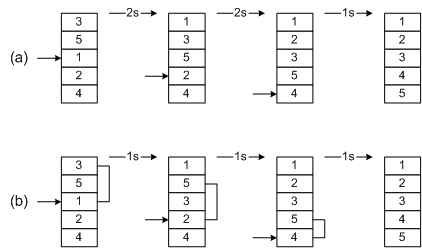# Shell Sort

Shell sort, developed by Donald L. Shell, is a non-stable in-place sort. Shell sort improves on the efficiency of insertion sort by quickly shifting values to their destination. Average sort time is O(n7/6), while worst-case time is O(n4/3). For further reading, consult Knuth .

## Theory

In Figure 2-2(a) we have an example of sorting by insertion. First we extract 1, shift 3 and 5 down one slot, and then insert the 1, for a count of 2 shifts. In the next frame, two shifts are required before we can insert the 2. The process continues until the last frame, where a total of 2 + 2 + 1 = 5 shifts have been made.

In Figure 2-2(b) an example of shell sort is illustrated. We begin by doing an insertion sort using aspacingof two. In the first frame we examine numbers 3-1. Extracting 1, we shift 3 down one slot for a shift count of 1. Next we examine numbers 5-2. We extract 2, shift 5 down, and then insert 2. After sorting with a spacing of two, a final pass is made with a spacing of one. This is simply the traditional insertion sort. The total shift count using shell sort is 1+1+1 = 3. By using an initial spacing larger than one, we were able to quickly shift values to their proper destination.Figure 2-2: Shell Sort

Various spacings may be used to implement a shell sort. Typically the array is sorted with a large spacing, the spacing reduced, and the array sorted again. On the final sort, spacing is one. Although the shell sort is easy to comprehend, formal analysis is difficult. In particular, optimal spacing values elude theoreticians. Knuth recommends a technique, due to Sedgewick, that determines spacinghbased on the following formulas:

hs= 9·2s- 9·2s/2+ 1, if s is even
hs= 8·2s- 6·2(s+1)/2+ 1, if s is odd

These calculations result in values (h0,h1,h2,…) = (1,5,19,41,109,209,…). Calculate h until 3ht >= N, the number of elements in the array. Then choose ht-1 for a starting value. For example, to sort 150 items, ht = 109 (3·109 >= 150), so the first spacing is ht-1, or 41. The second spacing is 19, then 5, and finally 1.

## Implementation in C

An ANSI-C implementation for shell sort is included. ```Typedef T``` and comparison operator `compGT` should be altered to reflect the data stored in the array. The central portion of the algorithm is an insertion sort with a spacing of h.

## Implementation in Visual Basic

A Visual Basic implementation for shell sort is included.